Precalculus : Sum and Difference Identities For Tangent

Example Questions

Example Question #1 : Sum And Difference Identities

In the problem below,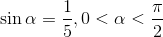and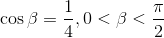.

Find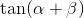.Explanation:

Since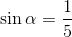and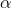is in quadrant I, we can say that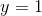and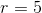and therefore: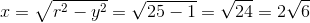So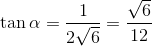.

Since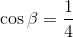and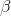is in quadrant I, we can say thatand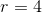and therefore: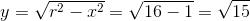.

So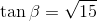Using the tangent sum formula, we see: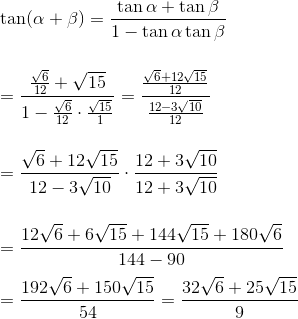Example Question #2 : Sum And Difference Identities

In the problem below,and.

Find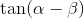.Explanation:

Sinceandis in quadrant I, we can say thatandand therefore:So.

Sinceandis in quadrant I, we can say thatandand therefore:So.

Using the tangent sum formula, we see: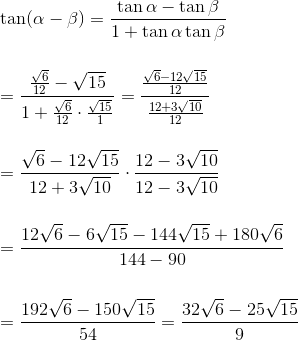Example Question #1 : Sum And Difference Identities

Given that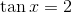and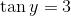, find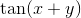.Explanation:

Jump straight to the tangent sum formula: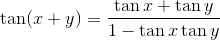From here plug in the given values and simplify.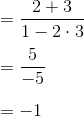Example Question #1 : Sum And Difference Identities

Which of the following expressions best represents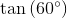?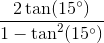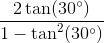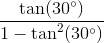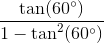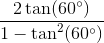Explanation:

Write the identity for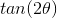.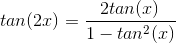Set the value of the angle equal to.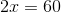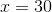Substitute the value ofinto the identity.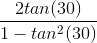All Precalculus Resources